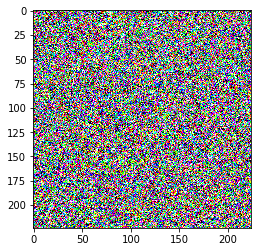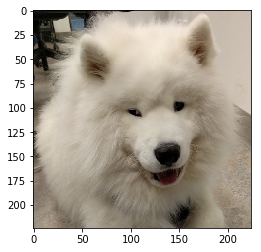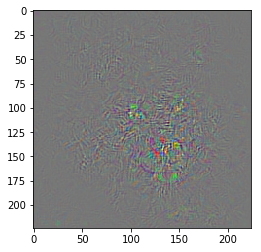# Optimizing the Input¶

This notebook demonstrates optimizing the inputs, and adversarial examples.

In :
import torch
import torch.nn as nn
import torch.nn.functional as F
import torch.optim as optim

from torchvision import datasets, transforms
import matplotlib.pyplot as plt
import torchvision.models
%matplotlib inline


We will study AlexNet as an example.

In :
import torchvision.models
alexnet = torchvision.models.alexnet(pretrained=True)


## Generating Data by Optimizing the Input¶

Our first experiment is to see whether we can generate the image of a Samoyed dog via backpropagation using AlexNet. In particular, we'll try to tune a random input so that AlexNet believes that the input is a Samoyed.

In :
# Let's try to create an image that AlexNet thinks is a Samoyed
target_label = 258 # Samoyed dog. From https://gist.github.com/yrevar/942d3a0ac09ec9e5eb3a


Since we will not be tuning AlexNet weights in this entire example, we will set the requires_grad parameter of AlexNet to False. This PyTorch setting means that PyTorch will not compute gradients for the parameters of AlexNet.

In :
alexnet.requires_grad = False # do not update AlexNet weights


Instead, we will be optimizing the input to AlexNet. Since AlexNet takes images of shape $3 \times 224 \times 224$, we will start with such a random image:

In :
# Initialize a random image
image = torch.randn(1, 3, 224, 224) + 0.5
image = torch.clamp(image, 0, 1)


Note that we set requires_grad = True for this input, because we do want PyTorch to be computing gradients, so we can optimize this input.

Actual optimization:

In :
# Use an optimizer to optimize the *input image*
optimizer = optim.Adam([image], lr=0.005)
criterion = nn.CrossEntropyLoss()

# Training:
for i in range(100):
out = alexnet(torch.clamp(image, 0, 1))
loss = criterion(out, torch.Tensor([target_label]).long())
loss.backward()
optimizer.step()
if i % 10 == 0:
target_prediction = torch.softmax(out, dim=1)[0, target_label]
print("Iteration %d, Loss=%f, target prob=%f" % (
i, float(loss), float(target_prediction)))

Iteration 0, Loss=8.903627, target prob=0.000136
Iteration 10, Loss=5.575039, target prob=0.003791
Iteration 20, Loss=3.999809, target prob=0.018319
Iteration 30, Loss=1.486968, target prob=0.226057
Iteration 40, Loss=0.619509, target prob=0.538209
Iteration 50, Loss=0.169662, target prob=0.843950
Iteration 60, Loss=0.058882, target prob=0.942818
Iteration 70, Loss=0.060514, target prob=0.941281
Iteration 80, Loss=0.009863, target prob=0.990185
Iteration 90, Loss=0.002597, target prob=0.997407


Now let's see the image of the fluffy dog:

In :
pltimg = torch.clamp(image, 0, 1).squeeze(0).transpose(0,1).transpose(1, 2).detach().numpy()
plt.figure()
plt.imshow(pltimg)

Out:
<matplotlib.image.AxesImage at 0x129914e10>That's a disappointment! What happened?!

Try a different class (e.g. 987: Corn). Also will fail...

## Gradient with respect to the input¶

We used this picture as an input to AlexNet last class. Let's try and compute the gradient of AlexNet with repsect to this input.

In :
import cv2
dog = cv2.resize(dog, (224, 224))
plt.imshow(dog)
dog = torch.Tensor(dog).transpose(0,2).transpose(1,2).unsqueeze(0)

torch.argmax(alexnet(dog))

Out:
tensor(258)Again, since we are computing the gradient with respect to this input, we need to set requires_grad=True.

In :
dog.requires_grad = True


Let's compute the gradient. Which pixels affect the prediction of our target (258 Samoyed) the most?

In :
criterion = nn.CrossEntropyLoss()
target_label = 258

out = alexnet(dog)
loss = criterion(out, torch.Tensor([target_label]).long())

dog_grad_np = dog_grad.transpose(0,1).transpose(1,2).numpy()

<matplotlib.image.AxesImage at 0x12d2f1a20>